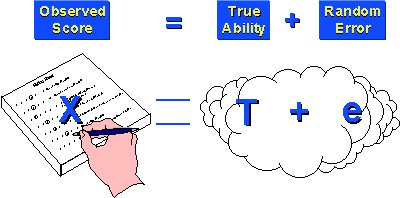True Score Theory

# True Score Theory

True Score Theory is a theory about measurement. Like all theories, you need to recognize that it is not proven – it is postulated as a model of how the world operates. Like many very powerful model, the true score theory is a very simple one. Essentially, true score theory maintains that every measurement is an additive composite of two components: true ability (or the true level) of the respondent on that measure; and random error. We observe the measurement – the score on the test, the total for a self-esteem instrument, the scale value for a person’s weight. We don’t observe what’s on the right side of the equation (only God knows what those values are!), we assume that there are two components to the right side.The simple equation of X = T + eX has a parallel equation at the level of the variance or variability of a measure. That is, across a set of scores, we assume that:

$$\textrm{var}(X) = \textrm{var}(T) + \textrm{var}(e_X)$$

In more human terms this means that the variability of your measure is the sum of the variability due to true score and the variability due to random error. This will have important implications when we consider some of the more advanced models for adjusting for errors in measurement.

Why is true score theory important? For one thing, it is a simple yet powerful model for measurement. It reminds us that most measurement has an error component. Second, true score theory is the foundation of reliability theory. A measure that has no random error (i.e., is all true score) is perfectly reliable; a measure that has no true score (i.e., is all random error) has zero reliability. Third, true score theory can be used in computer simulations as the basis for generating “observed” scores with certain known properties.

You should know that the true score model is not the only measurement model available. measurement theorists continue to come up with more and more complex models that they think represent reality even better. But these models are complicated enough that they lie outside the boundaries of this document. In any event, true score theory should give you an idea of why measurement models are important at all and how they can be used as the basis for defining key research ideas.